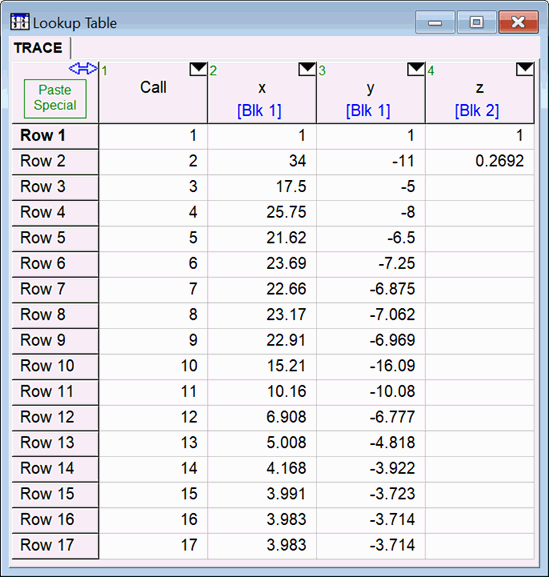Contents - Index

\$Trace

The \$Trace directive produces a table of the intermediate values for specified variables during the iteration process.  The \$Trace directive may be of use for debugging in situations in which the value of a variable is not converging as expected.  The format of the \$Trace command is

\$Trace /5000 X, Y, Z

The /5000 parameter is optional.  The number following the / character indicates the maximum number of iterations that will be recorded in the Trace table.  By default, this number is 1000.

X, Y, and Z are variables in the main section of the EES program.  Variables in Functions, Procedures, Modules, or Subprograms cannot be traced.

The \$Trace directive will place the intermediate values of the specified variables in a Lookup Table having the name TRACE.  If a Lookup table having the name TRACE exists, it will be deleted at the start of the calculations.  If you wish to keep the results of a Trace, rename the table.

\$Trace will be processed only when the Solve command is issued.  It will  have not effect when use with Solve Table or other commands in the Calculate menu.

It is best to place the \$Trace directive at the top of the program so that it is processed before any of the specified variables appear in equations.

Example: Trace the values of x, y, and z in the following system of equations

\$Trace x, y, z

x^3+y^2=77

x/(y^2+1)=x+y

z=x+y

After execution the following Lookup table will be produced.  Note that equations are solved in blocks.  The block structure is revealed in the Residuals window.  The block for each variable in the Trace table is shown below the block name.  Iterations for variables that are in different blocks cannot be directly compared as they are not occurring at the same time.